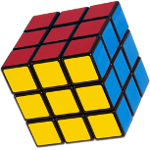### 3 x 3For solving this I started with the Rubik's beginner method, and have since switched to 2-look CFOP.

## Rubik's Method:

Step 1. F U R U'
Step 2. R' D' R D
Step 3.
a. U R U' R' U' F' U F
b. U' L' U L U F U' F'
Step 4. F R U R' U' F'
Step 5. R U R' U R U2 R'
Step 6. R U' L' U R' U' L
Step 7. R' D' R D

## 2-Look CFOP:

Plan the cross. No patterns for this, just make it in around 8 moves
F2L. Again no patterns to write down, just as you solve more you will figure out what works best
Edge OLL:
Dot Shape: F R U R' U' F' f R U R' U' f'
I Shape: F R U R' U' F'
L Shape: f R U R' U' f'
Corner OLL:
Anti Sune: R U2 R' U' R U' R'
Sune: R U R' U R U2 R'
H: R U R' U R U' R' U R U2 R'
L: F R' F' r U R U' r'
Pi: R U2 R2 U' R2 U' R2 U2 R
T: r U R' U' r' F R F'
U: R2 D R' U2 R D' R' U2 R'
PLL:
H: M2 U M2 U2 M2 U M2
T: R U R' U' R' F R2 U' R' U' R U R' F'
Ua: R U' R U R U R U' R' U' R2
Ub: R2 U R U R' U' R' U' R' U R'
Y: F R U' R' U' R U R' F' R U R' U' R' F R F'
Z: M' U M2 U M2 U M' U2 M2
Extra PLLs:
Ja: R U R' F' R U R' U' R' F R2 U' R'
Ra: R U' R' U' R U R D R' U' R D' R' U2 R'
E: R' U' F' R U R' U' R' F R2 U' R' U' R U R' U R

For center specific orientation, ex. picture cube, beginner method preserves the centers and CFOP will mix them up so you will have to resolve the centers after completing a solve with CFOP.
90° Counter Clockwise: M' U L' U' L U' L' U2 L U2 M
For clockwise do this pattern in reverse.
This pattern will scramble the top layer so you will need to repeat it 4 times or have an equal number of clockwise and counter clockwise rotations. Also when solving other centers after doing this pattern once do not disturb the U layer and use Dd twists to move to the next center.

180° Turns: M' U L' U2 L U L' U L U M
This pattern can be repeated 3 times to flip a single center 180° or similar to the 90° pattern an equal number of forward and backward times or 3 times total between 3 faces orienting with Dd turn.

Another option is to do this twice:
U R L U2 R' L'